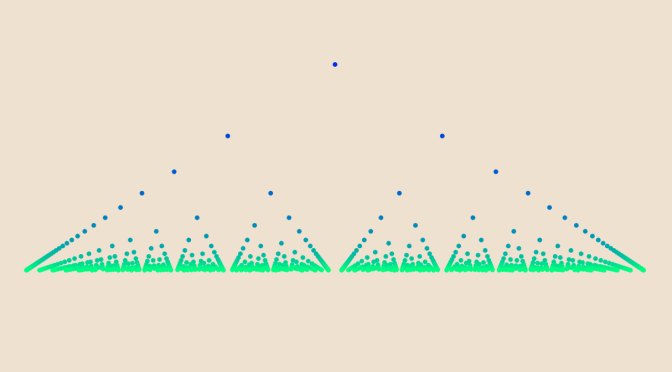# A function continuous at all irrationals and discontinuous at all rationals

Let’s discover the beauties of Thomae’s function also named the popcorn function, the raindrop function or the modified Dirichlet function.

Thomae’s function is a real-valued function defined as:
$f: \left|\begin{array}{lrl} \mathbb{R} & \longrightarrow & \mathbb{R} \\ x & \longmapsto & 0 \text{ if } x \in \mathbb{R} \setminus \mathbb{Q} \\ \frac{p}{q} & \longmapsto & \frac{1}{q} \text{ if } \frac{p}{q} \text{ in lowest terms and } q > 0 \end{array}\right.$

### $$f$$ is periodic with period $$1$$

This is easy to prove as for $$x \in \mathbb{R} \setminus \mathbb{Q}$$ we also have $$x+1 \in \mathbb{R} \setminus \mathbb{Q}$$ and therefore $$f(x+1)=f(x)=0$$. While for $$y=\frac{p}{q} \in \mathbb{Q}$$ in lowest terms, $$y+1=\frac{p+q}{q}$$ is also in lowest terms, hence $$f(y+1)=f(y)=\frac{1}{q}$$.

### $$f$$ right-sided and left-sided limits vanish at all points

We select $$x \in \mathbb{R}$$. For all $$\epsilon >0$$ one can find $$N > 1$$ integer with $$0 < \frac{1}{N} < \epsilon$$. Taking $$a= \sup \{c \in \mathbb{Z} \text{ | } c \le x N\}$$, we have $$x \in (\frac{a-1}{N},\frac{a+1}{N})$$. Now consider: \begin{aligned} A &=\{y \in \mathbb{Q} \setminus \{x\} \text{ | } y=\frac{r}{s} \in (\frac{a-1}{N},\frac{a+1}{N}),\\ & \ r \in \mathbb{Z}, \ 0 < s \le N, \ \gcd(r,s) = 1\} \end{aligned} $$A$$ is finite and by definition $$x \notin A$$. If $$A$$ is not empty, the distance $$D=d(x,A)$$ of $$x$$ to the set $$A$$ is defined and strictly positive. If $$A$$ is empty, we denote by $$B$$ the interval $$(\frac{a-1}{N},\frac{a+1}{N})$$ otherwise we take $$B=(\frac{a-1}{N},\frac{a+1}{N}) \cap (x-D,x+D)$$. In both cases, $$B$$ is an open interval containing $$x$$. By construction, $$B \setminus \{x\}$$ contains no rational number $$\frac{r}{s}$$ in lowest terms with $$s \le N$$. Hence for all $$z \in B \setminus \{x\}$$ we have $$0 \le f(z) < \frac{1}{N} < \epsilon$$ which had to be demonstrated.

### $$f$$ is discontinuous at all rational numbers

Consider $$x=\frac{p}{q}$$ in lowest terms. The sequence $$\displaystyle x_n=(1-\frac{1}{n \sqrt{2}})\frac{p}{q}$$ converges to $$x$$. For all $$n \ge 1$$ integer, $$x_n$$ is an irrational number as $$\sqrt{2}$$ is irrational, hence $$f(x_n)=0$$. Therefore $$\lim\limits_{n \to +\infty} f(x_n)=0$$ while $$f(x)=f(\frac{p}{q})=\frac{1}{q} > 0$$. More elegantly, we could have just notice that the irrational numbers are dense in $$\mathbb{R}$$. Or, we could have used previous paragraph stating that both $$f$$ left and right limits vanish.

### $$f$$ is continuous at all irrational numbers

Is clear as $$f$$ vanishes at all irrational points $$x$$ while both left and right $$f$$ limits vanish at all points.

### $$f$$ has a local maximum at all rational points

Consider a rational number $$a=\frac{p}{q}$$ in lowest terms. We have $$f(a)=\frac{1}{q}$$. As $$\lim\limits_{x \to a^-} f(x) = \lim\limits_{x \to a^+} f(x) = 0$$, for some $$\delta > 0$$ we have $$0 \le f(x) \le \frac{f(a)}{2}$$ when $$0 < \vert x-a \vert < \delta$$. Finally $$0 \le f(x) \le f(a)$$ for $$x \in (a - \delta, a + \delta)$$.

### $$f$$ is Lebesgue integrable

Follows from the fact that $$f$$ vanishes almost everywhere, the set of rational numbers having a Lebesgue measure equal to zero.

### $$f$$ is Riemann integrable on all intervals $$[a,b]$$

This is a consequence of Lebesgue’s integrability condition as $$f$$ is bounded (by $$1$$) and continuous almost everywhere. Or we can use the theorem stating that a regulated function is Riemann integrable.

### Python code I used to generate Thomae’s function image

import matplotlib.pyplot as plt import fractions as frac from math import log

 

points=[[p/float(q),1/float(q),log(float(q))] \ for q in range(1,50) for p in range(0,q+1) \ if frac.gcd(p,q) == 1] plt.axis('off') x,y,color=zip(*points) plt.scatter(x,y, c=color, s=3, edgecolor='none', cmap='winter') plt.savefig('D:tmp/to.png', dpi=350, facecolor='#EEE1D0')